# Selina solutions for Concise Mathematics Class 7 ICSE chapter 18 - Recognition of Solids (Representing 3-D in 2-D) [Latest edition]

#### Chapters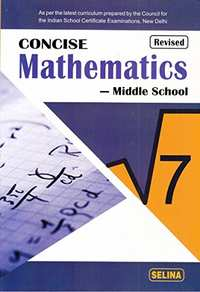## Chapter 18: Recognition of Solids (Representing 3-D in 2-D)

Exercise 18
Exercise 18

### Selina solutions for Concise Mathematics Class 7 ICSE Chapter 18 Recognition of Solids (Representing 3-D in 2-D) Exercise 18

Exercise 18 | Q 1.1

Identify if the following net can be used to form a cube: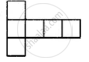• Yes

• No

Exercise 18 | Q 1.2

Identify if the following net can be used to form a cube: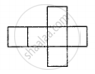• Yes

• No

Exercise 18 | Q 1.3

Identify if the following net can be used to form a cube: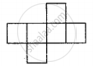• Yes

• No

Exercise 18 | Q 1.4

Identify if the following net can be used to form a cube: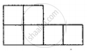• Yes

• No

Exercise 18 | Q 1.5

Identify if the following net can be used to form a cube: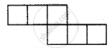• Yes

• No

Exercise 18 | Q 2

Draw at least three different nets for making a cube.

Exercise 18 | Q 3.1

The figure, given below, shows shadows of some 3D object when seen under the lamp of an overhead projector: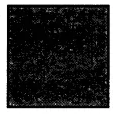A square

In this case, name the object.

Exercise 18 | Q 3.2

The figure, given below, shows shadows of some 3D object when seen under the lamp of an overhead projector: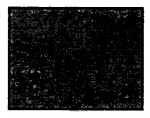A rectangle

In this case, name the object.

Exercise 18 | Q 3.3

The figure, given below, shows shadows of some 3D object when seen under the lamp of an overhead projector: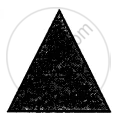A triangle

In this case, name the object.

Exercise 18 | Q 4

Using Euler’s formula, find the values of a, b, c and d.

 Faces a 5 20 6 Vertices 6 b 12 d Edges 12 9 c 12
Exercise 18 | Q 5.1

Dice are cubes with dots or dots on the face. Opposite faces of a die always have a total of seven on them.
In the given below net to make dice (cube), the numbers inserted in the square indicate the number of dots in it.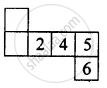Insert suitable numbers in the blank so that numbers in opposite faces of the die have a total of seven dots.

Exercise 18 | Q 5.2

Dice are cubes with dots or dots on the face. Opposite faces of a die always have a total of seven on them.
In the given below net to make dice (cube), the numbers inserted in the square indicate the number of dots in it.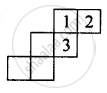Insert suitable numbers in the blank so that numbers in opposite faces of the die have a total of seven dots.

Exercise 18 | Q 6.1

The following figure represent the nets of some solid. Name the solid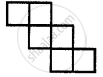Exercise 18 | Q 6.2

The following figure represent the nets of some solid. Name the solid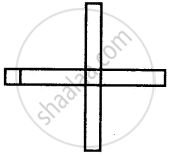Exercise 18 | Q 7

Draw a map of your classroom using proper scale and symbols for different objects.

Exercise 18 | Q 8

Draw a map of your school compound using proper scale and symbols for various features like a playground, main building, garden, etc.

Exercise 18 | Q 9

In the map of India, the distance between the two cities is 13.8 cm.
Taking scale: 1 cm = 12 km, find the actual distance between these two cities.

## Chapter 18: Recognition of Solids (Representing 3-D in 2-D)

Exercise 18## Selina solutions for Concise Mathematics Class 7 ICSE chapter 18 - Recognition of Solids (Representing 3-D in 2-D)

Selina solutions for Concise Mathematics Class 7 ICSE chapter 18 (Recognition of Solids (Representing 3-D in 2-D)) include all questions with solution and detail explanation. This will clear students doubts about any question and improve application skills while preparing for board exams. The detailed, step-by-step solutions will help you understand the concepts better and clear your confusions, if any. Shaalaa.com has the CISCE Concise Mathematics Class 7 ICSE solutions in a manner that help students grasp basic concepts better and faster.

Further, we at Shaalaa.com provide such solutions so that students can prepare for written exams. Selina textbook solutions can be a core help for self-study and acts as a perfect self-help guidance for students.

Concepts covered in Concise Mathematics Class 7 ICSE chapter 18 Recognition of Solids (Representing 3-D in 2-D) are Concept of Recognition of Solids, Concept for Mapping the Space Around Approximately Through Visual Estimation., Faces, Edges and Vertices.

Using Selina Class 7 solutions Recognition of Solids (Representing 3-D in 2-D) exercise by students are an easy way to prepare for the exams, as they involve solutions arranged chapter-wise also page wise. The questions involved in Selina Solutions are important questions that can be asked in the final exam. Maximum students of CISCE Class 7 prefer Selina Textbook Solutions to score more in exam.

Get the free view of chapter 18 Recognition of Solids (Representing 3-D in 2-D) Class 7 extra questions for Concise Mathematics Class 7 ICSE and can use Shaalaa.com to keep it handy for your exam preparation